# Measures - Teradata Warehouse Miner

## Teradata Warehouse Miner User Guide - Volume 3Analytic Functions

Product
Release Number
5.4.5
Published
February 2018
Language
English (United States)
Last Update
2018-05-04
dita:mapPath
yuy1504291362546.ditamap
dita:ditavalPath
ft:empty
dita:id
B035-2302
Product Category
Software

The four measurements made for association rules are support, confidence, lift and Z score.

## Support

Support is a measure of the generality of an association rule, and is literally the percentage (a value between 0 and 1) of groups that contain all of the items referenced in the rule. More formally, in the association rule defined as L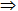R, L represents the items given to occur together (the Left side or antecedent), and R represents the items that occur with them as a result (the Right side or consequent). Support can actually be applied to a single item or a single side of an association rule, as well as to an entire rule. The support of an item is simply the percentage of groups containing that item.

Given the previous example of banking product ownership, let L be defined as the number of customers who own the set of products on the left side and let R be defined as the number of customers who own the set of products on the right side. Further, let LR be the number of customers who own all products in the association rule (note that this notation does not mean L times R), and let N be defined as the total number of customers under consideration. The support of L, R and the association rule are given by: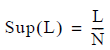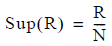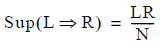Let’s say for example that out of 10 customers, 6 of them have a checking account, 5 have a savings account, and 4 have both. If L is (checking) and R is (savings), then Sup(L) is .6, Sup(R) is .5 and Sup(LR) is .4.

## Confidence

Confidence is the probability of R occurring in an item group given that L is in the item group. The equation to calculate the probability of R occurring in an item group given that L is in the item group is given by: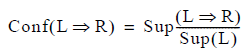Another way of expressing the measure confidence is as the percentage of groups containing L that also contain R. This gives the following equivalent calculation for confidence: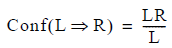Using the previous example of banking product ownership once again, the confidence that checking account ownership implies savings account ownership is 4/6.

The expected value of an association rule is the number of customers that are expected to have both L and R if there is no relationship between L and R. To say that there is no relationship between L and R means that customers who have L are neither more likely nor less likely to have R than are customers who do not have L.

The equation for the expected value of the association rule is: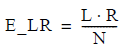An equivalent formula for the expected value of the association rule is: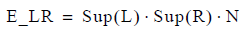Again using the previous example, the expected value of the number of customers with checking and savings is calculated as 6 * 5 / 10 or 3.

The expected confidence of a rule is the confidence that would result if there were no relationship between L and R. This simply equals the percentage of customers that own R, since if owning L has no effect on owning R, then it would be expected that the percentage of Ls that own R would be the same as the percentage of the entire population that own R. The following equation computes expected confidence: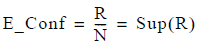From the previous example, the expected confidence that checking implies savings is given by 5/10.

## Lift

Lift measures how much the probability of R is increased by the presence of L in an item group. A lift of 1 indicates there are exactly as many occurrences of R as expected; thus, the presence of L neither increases nor decreases the likelihood of R occurring. A lift of 5 indicates that the presence of L implies that it is 5 times more likely for R to occur than would otherwise be expected. A lift of 0.5 indicates that when L occurs, it is one half as likely that R will occur. Lift can be calculated as follows: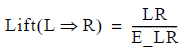From another viewpoint, lift measures the ratio of the actual confidence to the expected confidence, and can be calculated equivalently as either of the following: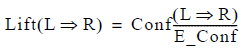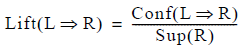The lift associated with the previous example of “checking implies savings” is 4/3.

## Z Score

Z score measures how statistically different the actual result is from the expected result. A Z score of zero corresponds to the situation where the actual number equals the expected. A Z score of 1 means that the actual number is 1 standard deviation greater than expected. A Z score of -3.0 means that the actual number is 3 standard deviations less than expected. As a rule of thumb, a Z score greater than 3 (or less than -3) indicates a statistically significant result, which means that a difference that large between the actual result and the expected is very unlikely to be due to chance. A Z score attempts to help answer the question of how confident you can be about the observed relationship between L and R, but does not directly indicate the magnitude of the relationship. It is interesting to note that a negative Z score indicates a negative association. These are rules LR where ownership of L decreases the likelihood of owning R.

The following equation calculates a measure of the difference between the expected number of customers that have both L and R, if there is no relationship between L and R, and the actual number of customers that have both L and R. It can be derived starting with either the formula for the standard deviation of the sampling distribution of proportions or the formula for the standard deviation of a binomial variable.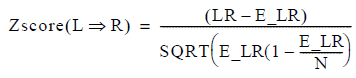or equivalently: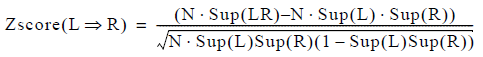The mean value is E_LR, and the actual value is LR. The standard deviation is calculated with SQRT (E_LR * (1 - E_LR/N)). From the previous example, the expected value is 6 * 5 / 10, so the mean value is 3. The actual value is calculated knowing that savings and checking accounts are owned by 4 out of 10 customers. The standard deviation is SQRT(3*(1-3/10)) or 1.449. The Z score is therefore (4 - 3) / 1.449 = .690.